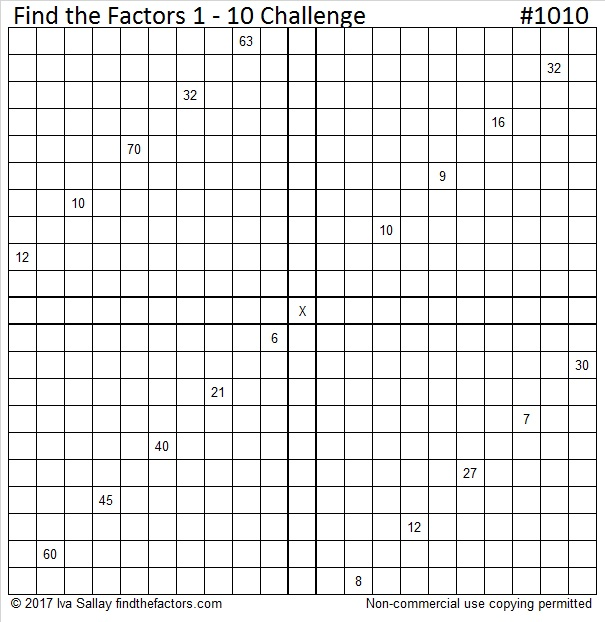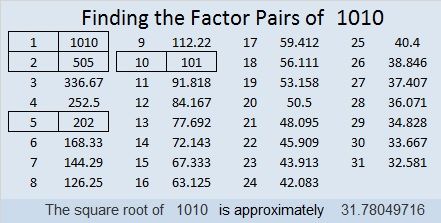# 1010 Find the Factors Challenge

Level 6 puzzles are difficult. At least they are until you’ve done a few, then they become much less difficult to solve.

This Find the Factors 1 – 10 Challenge is much more difficult. I published the first of these puzzles (#1000) last week, but I won’t necessarily make one every week. If you’ve done a few level 6 puzzles, see how you do with a Challenge Puzzle.Print the puzzles or type the solution in this excel file: 10-factors-1002-1011

Here’s a little about the number 1010:

1010 is the sum of two squares two different ways:
29² + 13² = 1010
31² + 7² = 1010

That means that 1010 is the hypotenuse of some Pythagorean triples:
200-990-1010 which is 10 times (20-99-101)
434-912-1010 calculated from 2(31)(7), 31² – 7², 31² + 7²
606-808-1010 which is (3-4-5) times 202
672-754-1010 calculated from 29² – 13², 2(29)(13), 29² + 13²

1010 is a fun-looking number in base 10.
It is also palindrome 262 in BASE 21 because 2(21²) + 6(21) + 2(1) = 1010

• 1010 is a composite number.
• Prime factorization: 1010 = 2 × 5 × 101
• The exponents in the prime factorization are 1, 1, and 1. Adding one to each and multiplying we get (1 + 1)(1 + 1)(1 + 1) = 2 × 2 × 2 = 8. Therefore 1010 has exactly 8 factors.
• Factors of 1010: 1, 2, 5, 10, 101, 202, 505, 1010
• Factor pairs: 1010 = 1 × 1010, 2 × 505, 5 × 202, or 10 × 101
• 1010 has no square factors that allow its square root to be simplified. √1010 ≈ 31.780497